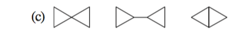# Problem: In each of the following groups of compounds, identify the one with the largest heat of combustion and the one with the smallest. In which cases can a comparison of heats of combustion be used to assess relative stability?

###### Problem Details

In each of the following groups of compounds, identify the one with the largest heat of combustion and the one with the smallest. In which cases can a comparison of heats of combustion be used to assess relative stability?What scientific concept do you need to know in order to solve this problem?

Our tutors have indicated that to solve this problem you will need to apply the Ring Strain concept. You can view video lessons to learn Ring Strain. Or if you need more Ring Strain practice, you can also practice Ring Strain practice problems.

What is the difficulty of this problem?

Our tutors rated the difficulty ofIn each of the following groups of compounds, identify the o...as medium difficulty.

How long does this problem take to solve?

Our expert Organic tutor, Jonathan took 1 minute and 33 seconds to solve this problem. You can follow their steps in the video explanation above.

What textbook is this problem found in?

Our data indicates that this problem or a close variation was asked in Organic Chemistry - Carey 8th Edition. You can also practice Organic Chemistry - Carey 8th Edition practice problems.